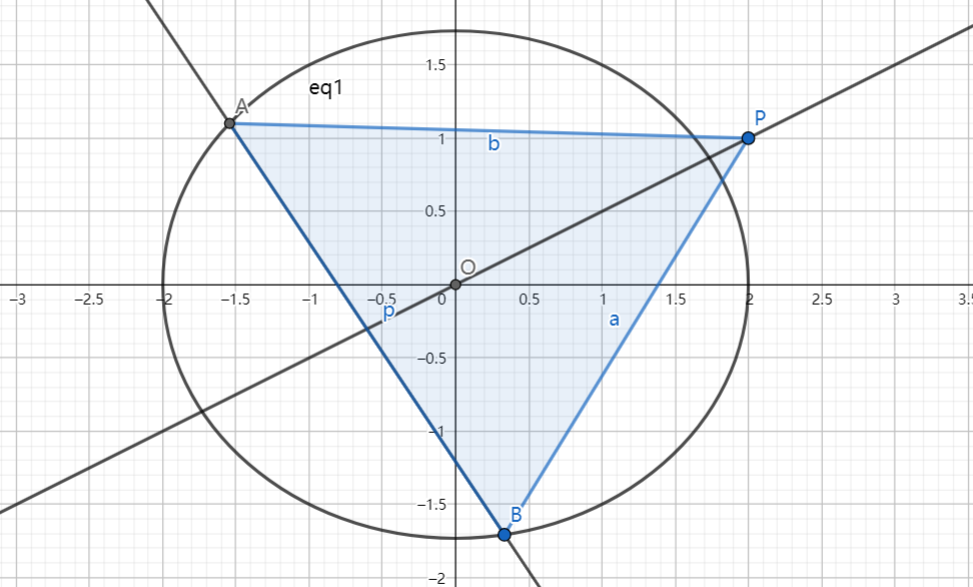# SAT1000 - P810

Geometry Level pendingAs shown above, the ellipse $C: \dfrac{x^2}{4}+\dfrac{y^2}{3}=1$, $O(0,0), P(2,1)$, line $l$ intersects with $C$ at point $A,B$, and line $OP$ bisects segment $AB$.

If the area of $\triangle APB$ reaches the maximum value, then find the equation of line $l$.

The equation of the line can be expressed as $y=kx+b$, submit $\lfloor 1000(k+b) \rfloor$.

Have a look at my problem set: SAT 1000 problems

×